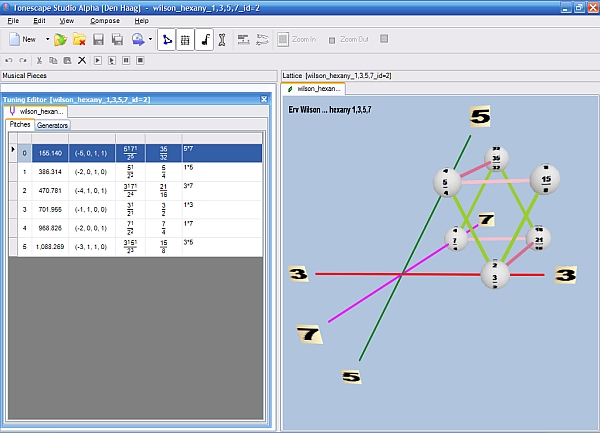# hexany

[John Chalmers, Divisions of the Tetrachord]

A six tone CPS generated by taking the elements or factors of a tetrad two at a time.

This set may be partioned into four pairs of inversionally related triads, each of which contains three of the four elements of the generating tetrad.

The Hexany has the interval sequence c b a b c d where a, b, c, d are not necessarily different intervals. d is the interval which completes the octave.

Hexanies are also defined in Equal temperaments.

The Stellated Hexany has 14 tones and is called both a "Mandala" and a Dekatesserany.

. . . . . . . . .
[Joe Monzo]

The calculation is made using factorials. The general formula for calculating the number of pitches p in a CPS composed of n odd-factors by finding all combinations of choosing m factors at a time is: p = n!/m!(n-m)!, therefore the formula for the eikosany is: p = 4!/2!(4-2)! = 24/2(2) = 24/4 = 6.

Below is a screenshot of a Tonescape® .tuning file of a 1,3,5,7 hexany. The notes are produced by multiplying together each pair of odd-factors.

"Octave"-equivalence (2:1 ratio) is assumed, thus, the Tonescape® .tuning file uses prime-factors 2, 3, 5, and 7, as shown in the Tuning Editor window on the left, but the Lattice window on the right does not show the inclusion of prime-factor 2.

(Download the Tonescape® .tuning file here. Click on the image for a high-resolution image.)The table below is ordered according to the column labeled "1357" which are the odd-factors, and makes it easy to see how the pitches of this tuning are chosen, using all possible combinations of 2 notes. A one ("1") signifies that that odd-factor is used, and a zero ("0") signifies that that odd-factor is not used.

```  2 3, 5 7-monzo  1357   ratio numerator  ~cents

<-1 1, 0 0>    =  1100 =  1 * 3 =   3  =   702.0
<-2 0, 1 0>    =  1010 =  1 * 5 =   5  =   386.3
<-2 0, 0 1>    =  1001 =  1 * 7 =   7  =   968.8
<-3 1, 1 0>    =  0110 =  3 * 5 =  15  =  1088.3
<-4 1, 0 1>    =  0101 =  3 * 7 =  21  =   470.8
<-5 0, 1 1>    =  0011 =  5 * 7 =  35  =   155.1
```

The table below puts the same notes in descending order of pitch:

```  2 3, 5 7-monzo  1357   ratio numerator  ~cents

<-3 1, 1 0>    =  0110 =  3 * 5 =  15  =  1088.3
<-2 0, 0 1>    =  1001 =  1 * 7 =   7  =   968.8
<-1 1, 0 0>    =  1100 =  1 * 3 =   3  =   702.0
<-4 1, 0 1>    =  0101 =  3 * 7 =  21  =   470.8
<-2 0, 1 0>    =  1010 =  1 * 5 =   5  =   386.3
<-5 0, 1 1>    =  0011 =  5 * 7 =  35  =   155.1
```
. . . . . . . . .

The tonalsoft.com website is almost entirely the work of one person: me, Joe Monzo. Please reward me for my knowledge and effort by choosing your preferred level of financial support. Thank you.

 support level donor: \$5 USD friend: \$25 USD patron: \$50 USD savior: \$100 USD angel of tuning: \$500 USD microtonal god: \$1000 USD